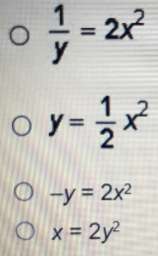# Which equation can be simplified to find the inverse of y=2x^2?

Which equation can be simplified to find the inverse of y=2x^2?